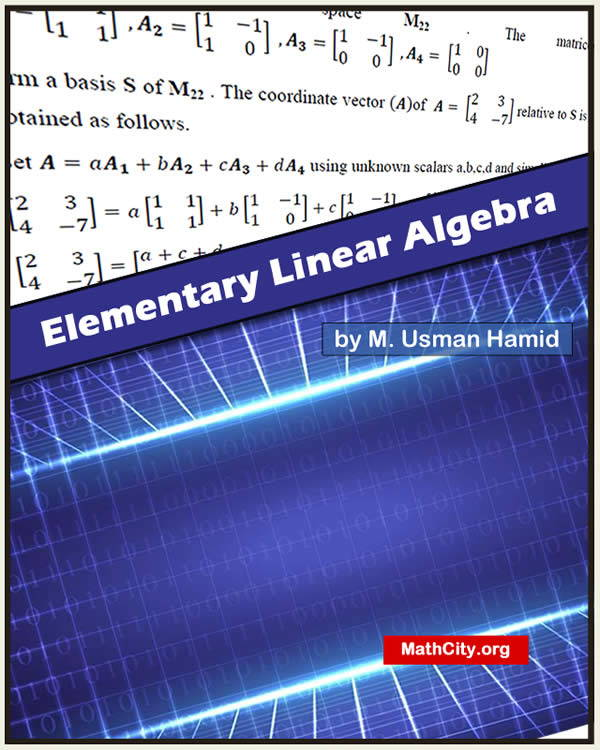# Elementary Linear Algebra by Muhammad Usman HamidLinear Algebra is the study of vectors and linear transformations. The main objective of this course is to help students learn in rigorous manner, the tools and methods essential for studying the solution spaces of problems in mathematics, engineering, the natural sciences and social sciences and develop mathematical skills needed to apply these to the problems arising within their field of study; and to various real world problems.

Our sincere gratitude goes out to Muhammad Usman Hamid for providing these notes and for making the effort to post them on MathCity.org.

 Name Elementary Linear Algebra Muhammad Usman Hamid 293 pages PDF (see Software section for PDF Reader) 4.38 mB
• System of Linear Equations: Representation in matrix form, matrices, operations on matrices, echelon and reduced echelon form, inverse of a matrix (by elementary row operations), solution of linear system, Gauss-Jordan method, Gaussian elimination.
• Vector Spaces: Definition and examples, subspaces. Linear combination and spanning set. Linearly Independent sets. Finitely generated vector spaces. Bases and dimension of a vector space. Operations on subspaces, Intersections, sums and direct sums of subspaces. Quotient Spaces.
• Inner product Spaces: Definition and examples. Properties, Projection. Cauchy inequality. Orthogonal and orthonormal basis. Gram Schmidt Process.
• Determinants: Permutations of order two and three and definitions of determinants of the same order. Computing of determinants. Definition of higher order determinants. Properties. Expansion of determinants.
• Diagonalization, Eigen-values and eigenvectors
• Linear mappings: Definition and examples. Kernel and image of a linear mapping. Rank and nullity. Reflections, projections, and homotheties. Change of basis. Theorem of Hamilton-Cayley.

Books Recommended:

• Curtis C. W., Linear Algebra
• Apostol T., Multi Variable Calculus and Linear Algebra.
• Anton H., Rorres C., Elementary Linear Algebra: Applications Version
• Dr. Karamat Hussain, Linear Algebra
• Linear Algebra by Seymour Lipschutz

• notes/elementary-linear-algebra-m-usman-hamid